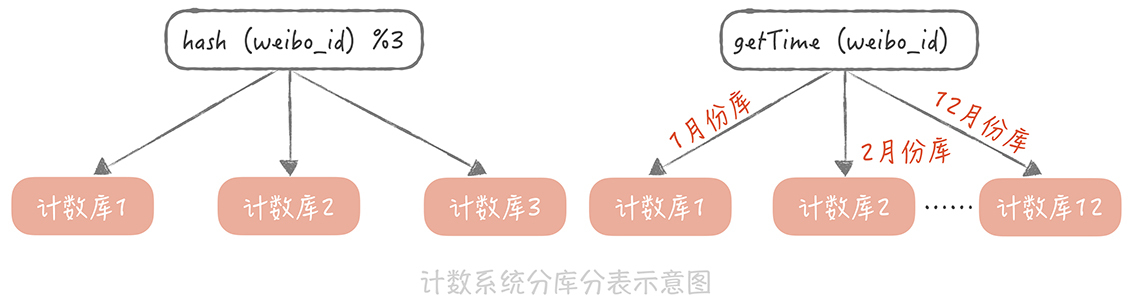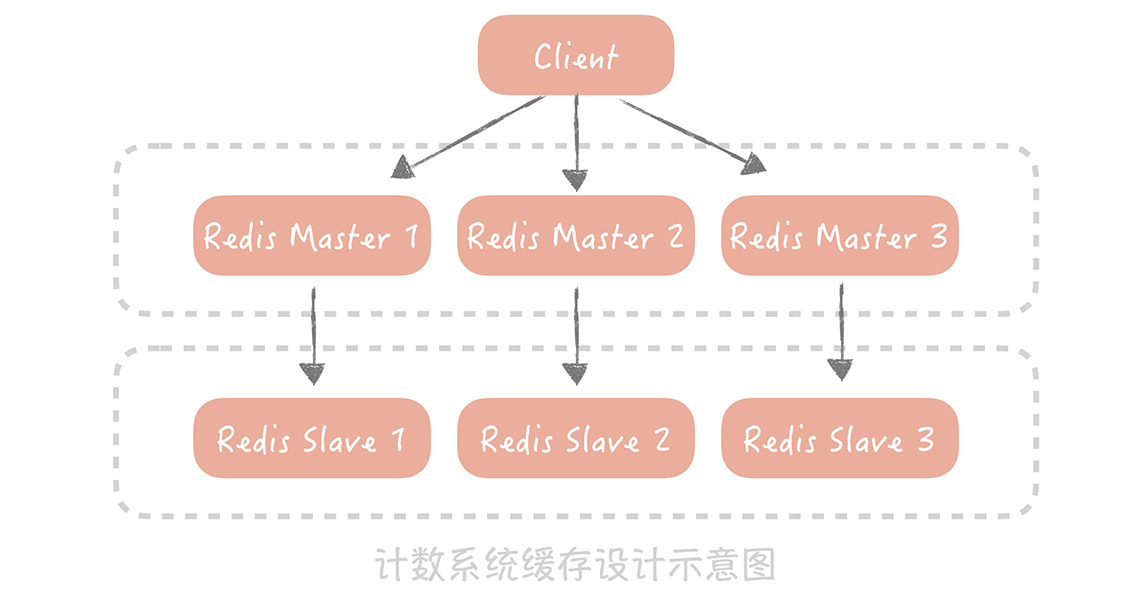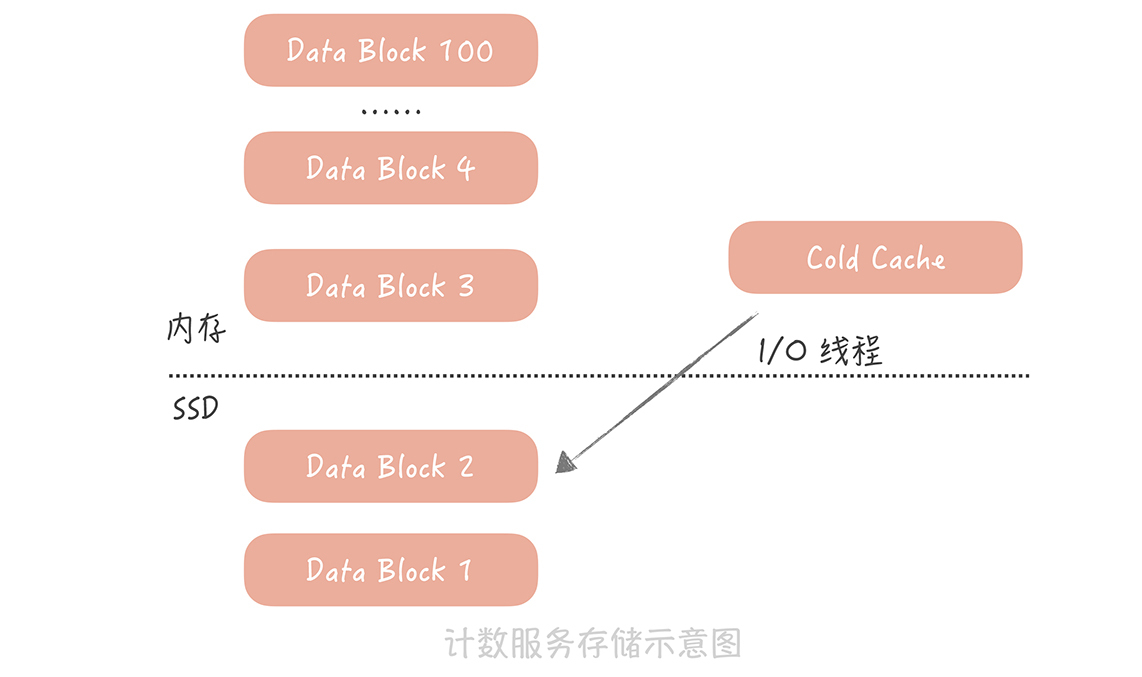37 计数系统设计（一）：面对海量数据的计数器要如何做？

支撑高并发的计数系统要如何设计

select repost_count, comment_count, praise_count, view_count from t_weibo_count where weibo_id = ?UPDATE t_weibo_count SET repost_count = repost_count + 1 WHERE weibo_id = 1;

UPDATE t_weibo_count SET repost_count = repost_count + 1 WHERE weibo_id = 1;

UPDATE  t_weibo_count SET repost_count = repost_count +1 WHERE weibo_id = 1;

UPDATE t_weibo_count SET repost_count = repost_count + 3 WHERE weibo_id = 1;

如何降低计数系统的存储成本

h1 = hash1(weibo_id) // 根据微博 ID 计算 Hash

h2 = hash2(weibo_id) // 根据微博 ID 计算另一个 Hash，用以解决前一个 Hash 算法带来的冲突

for s in 0,1000

pos = (h1 + h2*s) % tsize // 如果发生冲突，就多算几次 Hash2

if(isempty(pos) || isdelete(pos))

t[ pos ] = item  // 写入数组

for s in 0,1000

pos = (h1 + h2*s) % tsize  // 依照插入数据时候的逻辑，计算出存储在数组中的位置

if(!isempty(pos) && t[pos]==weibo_id)

return t[pos]

return 0

insert(FFFF) // 插入一个特殊的标